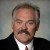# How Is the Defects Per Million Opportunities (DPMO) to Sigma Level and Yield Conversion Chart Determined?

ByDr. Mikel Harry

We must understand the sigma of a process is merely an equivalent unilateral figure-of-merit. As such, it is always regarded (and reported) as a short-term measure of capability. The metric can be directly computed when continuous data is at hand, or it can be statistically synthesized from discrete data. In the latter context, a reported sigma is only analogous to the Z statistic. In other instances, the sigma metric can be made to represent the normalized capability of several critical-to-quality characteristics (CTQs). To better facilitate our understanding, consider the following example.

For the first scenario, we will postulate a single random normal CTQ with a symmetrical bilateral performance specification. Respectively, we compute Z.usl.st = (USL – T) / S.st = +3.5 and Z.lsl.st = (LSL – T ) / S.st = -3.5. Of course, each Z must be transformed into a tail-area probability (by way of a table or Excel macros). Doing so reveals that p(Z.usl.st) = 1 – normsdist(Z.usl.st) = 1 – normsdist(+3.5) = 1 – .99976733 = .00023267. On the other end, we compute p(Z.lsl.st) = normsdist(Z.lsl.st) = normsdist(-3.5) =.00023267.

Combining both probabilities exposes the total probability of defect. Doing so reveals 00023267 + .00023267 = .00046535. Given this, the short-term first-time yield was presented as Y.ft.st = 1 – .00046535 = .99953465. With this as a backdrop, the equivalent unilateral capability was given as Z.bench = Z.st = normsinv(Y.ft.st) = normsinv(.99953465) = 3.31071537. In rounded form, we accept the quantity 3.31 Sigma. Consequently, the equivalent long-term unilateral capability can be approximated as Z.lt = Z.st – 1.50 = 3.31 – 1.50 = 1.81. Translated, this is equivalent to DPMO = 35,148.

Handpicked Content:   How Do I Determine Defects Per Million (DPM) for Sigma Values Greater Than 6 (3.4 DPM)?

Let us now postulate a second application scenario. Suppose we examined a certain discrete CTQ and discovered the long-term defect rate to be DPMO = 4,200. Converting this quality metric to an equivalent long-term first-time yield discloses the approximation Y.ft.lt = 1- (DPMO / 10^6) = 1- (4,200 / 10^6) = 1 – .00420 = .99580. Of course, such a long-term first-time yield can be statistically converted to an “equivalent” long-term unilateral standard normal deviate. So doing provides the quantity Z.lt = normsinv(1 – Y.ft.lt) = normsinv(.99580) = 2.63626982, or 2.64 in rounded form. Since no short-term information or data were available, the instantaneous (short-term) capability was approximated as Z.bench = Z.st = Z.lt + 1.5 = 2.64 + 1.50 = 4.14. Thus, we assert the process has an inherent capability of 4.14 Sigma.

Pooling the two scenarios, we must convert the respective sigmas into first-time yield values. For the first scenario, we have Y.ft.st.1 = .99953465. For the second scenario, we observe Y.ft.st.2 = .99998234. The short-term rolled-throughput yield would then be computed as Y.rt.st = Y.ft.st.1 * Y.ft.st.2 = .99953465 * .99998234 = .99951700. The normalized yield would be given as Y.norm = Y.rt.st^(1/K) = .99951700^(1/2) = .99975847.

Converting Y.norm to an equivalent unilateral short-term Z value reveals Z.norm.st = 3.49, meaning that the overall sigma of the process should be reported as 3.49. The normalized long-term unilateral capability was approximated as Z.norm.lt = Z.norm.st – 1.50 = 3.49 – 1.50 = 1.99. Interestingly, such a level of capability translates to a quality level of DPMO = 23,293. Thus, we are able to merge heterogeneous metrics for the purpose of quality reporting.

##### Dr. Mikel Harry

1.Omar

Information given here is either wrong / print mistake…

Article: “How is the defects per Million opportunities (DPMO) to sigma level and Yield conversion chart determined?”

Para # 2 >> from Z.short term >> you got the DPMO
Para # 3 >> from same DPMO >> you calculated Z.short term

But, the numbers are not matching –>
Para # 2 >> it is shown as 3.5
Para # 3 >> it is shown as 3.31

How is that possible?

2.Mary McShane Vaughn

The Z = 3.5 is two-sided, wherein P(Z3.5) = 0.00023267. Here we are assuming a normally distributed quality characteristic. In the example, it is implied that the process is centered on spec nominal, but this does not have to be the case. We basically are finding the fraction out of spec at this point, and z is our means to do so.

The sigma level is a one-sided metric, so we find the z that satisfies P(Z>z)=0.00023267. This is equal to 3.31. This is the reported sigma level.

I agree it is strange, and actually, process capability measures like Cpk or Cpm probably are more informative.

3.Mary McShane Vaughn

Sorry, the text did not print out correctly: in first line, I meant Pr(Z3.5) = 0.00023267.